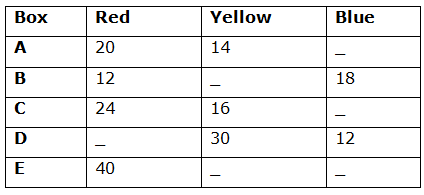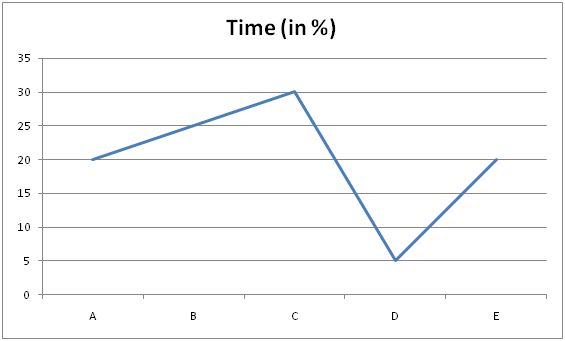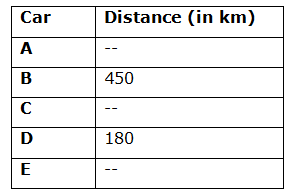# SBI PO Quantitative Aptitude Questions 2019 (Day-16) High Level New Pattern

SBI PO 2019 Notification is about to come and it is the most awaited exam among the aspirants. We all know that new pattern questions are introducing every year in the SBI PO exam. Further, the questions are getting tougher and beyond the level of the candidate’s expectations.

Our IBPS Guide is providing High-Level New Pattern Quantitative Aptitude Questions for SBI PO 2019 so the aspirants can practice it on a daily basis. These questions are framed by our skilled experts after understanding your needs thoroughly. Aspirants can practice these high-level questions daily to familiarize with the exact exam pattern. We wish that your rigorous preparation leads you to a successful target of becoming SBI PO.

[WpProQuiz 5080]

### Click Here for SBI PO Pre 2019 High-Quality Mocks Exactly on SBI Standard

Direction (1- 5): Study the following information carefully and answer the given questions

The given table shows the five different boxes contain three different color balls.1) Probability of drawing one blue from box E is 1/4 and the probability of drawing one red ball from box E is 2/15. The probability of drawing one yellow ball from box D is 5/12. Find the sum of the probabilities of drawing one yellow ball from box E and one red ball from box D?

a) 31/30

b) 53/62

c) 61/52

d) 73/67

e) None of these

2) The probability of drawing one yellow ball from box A is 2/7. Probability of drawing one red ball from box B is 3/10. Find the sum of the probability of drawing 2 blue balls from box A and the probability of drawing two yellow balls from box B?

a) 137/728

b) 127/728

c) 107/728

d) 117/728

e) None of these

3) If the probability of one red ball drawing from box A is 1/3 and the probability of drawing either a Yellow or Red ball from box C is 4/5, and then find the difference between the probability of drawing 2 balls from box A such that both balls are either yellow or Red and the probability of drawing three balls from box C such that 2 of them are blue balls and one of them is red ball?

a) 899/8673

b) 899/6873

c) 899/8373

d) 879/8763

e) None of these

4) The probability of drawing a Yellow ball from box E is 2/15 and the probability of drawing a blue ball from box E is 1/5.

Quantity I: The number of total balls in Box F is 5/6 times the total number of balls in Box E. The number of red balls from box F is 6 less than the number of Yellow balls from box F and the number of Blue balls from box F is 2/3 times the number of blue balls in Box E. Find the number of red balls in Box F?

Quantity II: The number of Red balls in Box G is 9 more than the half of the total number of Red balls in E. The number of Yellow balls from box G is same as the number of yellow balls in Box D. The probability of drawing a blue ball from box G is 11/70. Find the number of blue balls in Box G?

a) Quantity I > Quantity II

b) Quantity I < Quantity II

c) Quantity I ≤ Quantity II

d) Quantity I ≥ Quantity II

e) Quantity I = Quantity II (or) Relationship cannot be determined.

5) The probability of getting one blue ball from Box A is 3/20 and the probability of getting one red ball from box D is 3/10. Total number of balls in Box A is what percentage more/less than the total number of balls in Box D?

a) 55.55% less

b) 66.67% more

c) 44.44% less

d) 22.22% more

e) 33.33% less

Direction (6 – 10): Study the following information carefully and answer the given questions:

The given line graph shows the percentage of time taken by five different cars in the journey.

Total time taken in the journey= 60 hoursThe given table shows the distance covered by car.6) If the train crosses the car A running in the same direction in 36 seconds and the same train crosses the car E running the opposite direction in 6 seconds. Train runs at the speed of 60 kmph and also crosses the pole in 9 seconds.  What is the difference between the distance covered by car A and car E?

a) 180 km

b) 220 km

c) 200 km

d) 150 km

e) None of these

7) A train running at the speed of 72 kmph crosses another train in opposite direction at 54 kmph in 12 seconds. The first train crosses the 260m long bridge in 20 seconds and the second train crosses car C running in the opposite direction in 6 seconds, then find the distance covered by car C?

a) 2052 km

b) 1082 km

c) 2064 km

d) 2084 km

e) None of these

8) A train can travel 50% faster than a car A. Both start from Chennai at the same time and reach Bangalore 150 km away from Chennai at the same time. On the way, however, the train lost about 25 minutes while stopping at the stations. If the speed of Car A, Car C and Car E in the ratio of 6:4:3 then find the average of the distance covered by all the five cars together?

a) 846 km

b) 746 km

c) 946 km

d) 646 km

e) None of these

9) The sum of the speed of car B and car D is what percent of the distance covered by car C, if the speed of car C is 40kmph?

a) 15.25%

b) 10.75%

c) 12.5%

d) 8%

e) None of these

10) If the total distance covered by all the car is 1350 km and the distance covered by car A, car C and car E in the ratio of 2 : 3 : 1. What is the average speed of all the cars together?

a) 28 kmph

b) 25 kmph

c) 26 kmph

d) 24 kmph

e) None of these

Direction (1-5) :

Number of yellow balls from box E=x

Number of blue balls from box E=y

Total number of balls from box E=40+x+y

Number of Red balls from box D=z

Total number of balls from box D = z+30+12=z+42

y/(40 + x + y) = 1/4

40+x+y=4y

X – 3y + 40=0 ——– (1)

40/(40+x+y) = 2/15

600=80+2x+2y

2x+2y-520=0

x+y-260 =0 ———- (2)

By substituting 1 and 2, we get,

-4y=-300

Y=75

X – (3)*75+40=0

x=185

Total number of balls from box E=185+75+40=300

30/(z+42)=5/12

360 = 5z+210

5Z=150

Z = 30

Total balls from box D=30+42=72

Required sum=185C1/300C1 + 30C1/72C1

= > (37 + 25)/60 = 62/60 = 31/30

Number of blue balls from box A=x

Total balls from box A = x+20+14 = x+34

14/(x+34) = 2/7

98 = 2x+68

2x = 30

x=15

Total balls from box A=15+34=49

Number of yellow balls from box B=y

Total balls from box B=y+12+18=y+30

12/(y+30)=3/10

3y+90=120

Y=10

Total balls from box B=30+10=40

Required sum=15C2/49C2 + 10C2/40C2

= > 5/56 + 3/52 = (260+168) /2912

= > 107/728

Number of blue balls from box A=x

20C1/(x+34)C1 = 1/3

20/(x+34) = 1/3

X+34=60

x=26

Total balls from box A=26+34=60

Number of blue balls from box C=y

24C1/(y+40)C1 + 16C1/(y+40)C1= 4/5

40/(y+40)=4/5

200=4y+160

y=10

Total balls from box C=10+24+16=50

2 red balls from box A=20C2=20*19/2=190

2 yellow balls from box A=14*13/2=91

Total number of possible=60C2=60*59/2=1770

Required probability=281/1770

From box C = (10C2*24C1) / 50C3

=1080/19600

=27/490

Required difference=281/1770 – 27/490

=89900/867300

=899/8673

Number of yellow balls from box E=x

Number of Blue balls from box E=y

X / (x+y+40) = 2/15

15x=2x+2y+80

13x – 2y=80 ——- (1)

Y / (x+y+40) = 1/5

x+y+40=5y

x – 4y + 40 = 0 ——– (2)

(1)*2 – (2)

25x=200

x=8

-4y=-40-8

y=12

Total number of balls from box E=12+8+40=60

Quantity I: The number of total balls in Box F is 5/6 times the total number of balls in Box E. The number of red balls from box F is 6 less than the number of Yellow balls from box F and the number of Blue balls from box F is 2/3 times the number of blue balls in Box E. Find the number of red balls in Box F?

Total number of balls from box F= (5/6)*60=50

Number of blue balls from box F= (2/3)*12=8

Number of red balls from box F=z

Number of yellow balls from F=z+6

Z+z+6+8=50

2z=36

Z=18 balls

Yellow balls from F=24

The total number of red balls in Box F (z) = 18 balls

Quantity II: The number of Red balls in Box G is 9 more than the half of the total number of Red balls in E. The number of Yellow balls from box G is same as the number of yellow balls in Box D. The probability of drawing a blue ball from box G is 11/70. Find the number of blue balls in Box G?

Red balls from box G = (40/2) + 9 = 29 balls

Yellow balls from G = 30

Number of blue balls from G=b

b / (30+29+b) = 11/70

70b=649 + 11b

59b=649

b=11

The total number of blue balls in Box G (b) = 11 balls

Hence, Quantity I > Quantity II

Probability of one blue ball from Box A = xC1/(20+14+x)C1= 3/20

x/(34 + x) = 3/20

20x = 102 + 3x

17x = 102

X = 6

Total number of balls in Box A = 34+6 = 40

Probability of one red ball from Box D = yC1 / (30+12+y)C1 = 3/10

y/(42+y)= 3/10

10y = 42*3 + 3y

7y = 42 * 3

Y = 3*6 = 18

Total number of balls in Box D = 42+18 = 60

Required percentage = [(60-40)/60] * 100

= > (20/60)*100 = (1/3)*100 = 33.33 %

Direction (6-10) :

Length of the train=x

Speed of car A=y

Speed of car E=z

x=60*(5/18)*9

x=150 m

150 = (60 – y)*(5/18)*36

Y=45 kmph

150 = (60+z)*(5/18)*6

90=60+z

Z=30 kmph

Time of car A = (20/100)*60 =12 hours

Distance covered by car A=12*45=540 km

Time taken by car E = (20/100)*60 = 12 hours

Distance covered by car E=12*30=360 km

Required difference=540 – 360 =180 km

Length of first train=x

Length of second train=y

Speed of car C=s

X+260 = 72*(5/18)*20

x=400-260

x=140 m

140+y = (72+54)*(5/18)*12

140+y = 420

Y=280 m

280=(s+54)*(5/18)*6

S=114 kmph

Time taken by car C = (30/100)*60=18 hours

Distance covered by car C =18*114=2052 km

Speed of car A=x

Speed of train= (150/100)*x=3x/2

150/x – 150/(3x/2) = 25/60

X=120 kmph

Speed of car C = (4/6)*120=80 kmph

Speed of car E= (3/6)*120=60 kmph

Time taken by car A= (20/100)*60=12 hours

Time taken by car C= (30/100)*60=18 hours

Time taken by car E= (20/100)*60=12 hours

Distance covered by car A=12*120=1440 km

Distance covered by car C=18*80=1440 km

Distance covered by car E=60*12=720 km

Average distance = (1440+1440+720+450+180)/5=846 km

Time taken by car B= (25/100)*60 =15 hours

Time taken by car D= (5/100)*60 =3 hours

Speed of car B= 450/15=30 kmph

Speed of car D=180/3=60 kmph

Time taken by car C= (30/100)*60=18

Distance covered by car C=40*18=720 km

Required percentage = [(30+60)/720] * 100=12.5%

Time taken by car A= (20/100)*60=12hours

Time taken by car B= (25/100)*60=15 hours

Time taken by car C= (30/100)*60=18 hours

Time taken by car D= (5/100)*60=3 hours

Time taken by car E= (20/100)*60=12 hours

Distance covered by car B and D =450+180=630

Remaining distance=1350 – 630=720 km

Distance covered by car A= (2/6)*720=240 km

Distance covered by car C= (3/6)*720=360 km

Distance covered by car E= (1/6)*720=120 km

Speed of car A=240/12=20 kmph

Speed of car B=450/15=30 kmph

Speed of car C=360/18=20 kmph

Speed of car D=180/3=60 kmph

Speed of car E=120/12=10 kmph

Average speed= (20+30+20+60+10)/5=28 kmph

### Click Here for SBI PO Pre 2019 High-Quality Mocks Exactly on SBI Standard